Categories

# And basic image processing operations (Python)

PIL provides a common image processing functions, and a large number of basic image manipulation, such as image scaling, cropping, rotation, color conversion and the like.

Matplotlib provides a powerful drawing capabilities, under the pylab / pyplot interface contains many user-friendly functions to create the image.

In order to observe and further processing of image data, you first need to load an image file, and to view the image data, we need to draw it out.

``````from PIL import Image
import matplotlib.pyplot as plt
import numpy as np

# 加载图像
img = Image.open("tmp.jpg")
# 转为数组
img_data = np.array(img)
# 可视化
plt.imshow(img_data)
plt.show()``````

For images, we have a common operation to resize the image, rotating the image and the gradation transformation

``````from PIL import Image
import matplotlib.pyplot as plt

img = Image.open("girl.jpg")

plt.figure()
# 子图
plt.subplot(221)
# 原图
plt.imshow(img)
plt.subplot(222)
# 将图像缩放至 256 * 256
plt.imshow(img.resize((256, 256)))
plt.subplot(223)
# 将图像转为灰度图
plt.imshow(img.convert('L'))
plt.subplot(224)
# 旋转图像
plt.imshow(img.rotate(45))
# 保存图像
plt.savefig("tmp.jpg")
plt.show()``````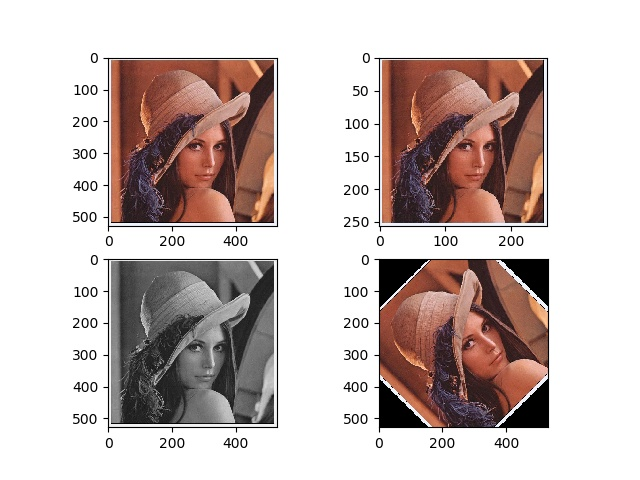In normal use, the contour drawing of the image are often used because the same is applied to draw the profile as a threshold value for each coordinate (x, y) is required, it is necessary to image grayscale

``````from PIL import Image
import matplotlib.pyplot as plt
import numpy as np

img = Image.open("girl.jpg")

gray_img = np.array(img.convert('L'))
plt.figure()
# 绘制图像灰度化
plt.gray()
# 关闭坐标轴
plt.axis('off')
# 绘制灰度图像
plt.contour(gray_img, origin='image')
plt.figure()
# 绘制直方图,flatten()表示将数组展平
plt.hist(gray_img.flatten(), 128)
plt.show()
``````

Contour plot and histogram: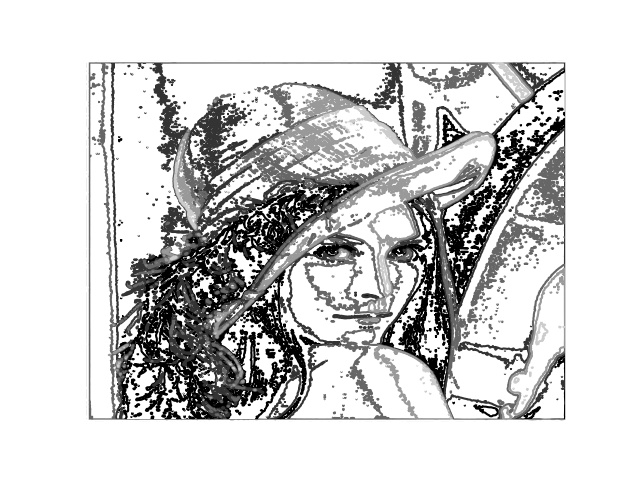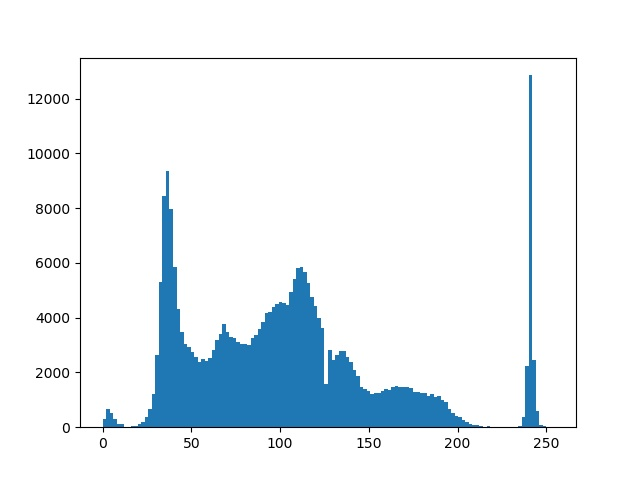Histogram of an image used to characterize the distribution of the pixel values ​​of the image. With a number of inter-cell range of pixel values ​​to specify characterized, inter fall within each cell will be represented by the number of pixels between the cell range. a hist () function for drawing an image histogram, which accept only one-dimensional array as the first input parameter, the second parameter which specifies the number of inter-cell.

Sometimes users and applications need to interact, as a marker at some point in the image. ginput Pylab / pyplot library () function can be achieved interactively mark

``````from PIL import Image
import matplotlib.pyplot as plt

img = Image.open(r"girl.jpg")
plt.imshow(img)
x = plt.ginput(3)
print("clicked point: ", x)``````

Note: This interaction Integrated Environment (pyCharm) If you can not bring up an interactive window can not be clicked, can successfully execute the command window.

Above we () function will be converted into an Image object numpy array by the array, the following will show how to convert from an array to Image object

``````from PIL import Image
import numpy as np

img = Image.open(r"girl.jpg")
img_array = np.array(img)
img = Image.fromarray(img_array)``````

A useful example of the gradation conversion in an image is histogram equalization. Histogram equalization is a grayscale histogram of the image a flattened, so that a probability distribution for each gradation value in the transformed image are the same. Histogram equalization is usually a very good method of image intensity is normalized, and the contrast of the image can be enhanced.

Histogram equalization transform function is the cumulative distribution function of pixel values ​​in the image (cumulative distribution function, to map the range of pixel values ​​to normalized target range operation).

``````from PIL import Image
import matplotlib.pyplot as plt
import numpy as np

def histogram_equalization(img: np, nbr_bins=256):
imhist, bins = np.histogram(img.flatten())
cdf = imhist.cumsum() # 累计分布函数
# 归一化
cdf = 255 * cdf / cdf[-1]
# 使用累积分布函数进行线性插值，计算新的像素值
img2 = np.interp(img.flatten(), bins[:-1], cdf)
return img2.reshape(img.shape), cdf

img = Image.open(r"girl.jpg").convert('L')
img2, cdf = histogram_equalization(np.array(img))
plt.figure()
plt.gray()
# 绘制子图
plt.subplot(232)
# 变换函数
plt.plot(cdf)
plt.subplot(231)
plt.hist(np.array(img).flatten(), 256)
# 关闭坐标轴，对上一个子图有效
plt.axis('off')
plt.subplot(233)
plt.hist(np.array(img2).flatten(), 256)
plt.axis('off')
plt.subplot(234)
plt.imshow(img)
plt.axis('off')
plt.subplot(236)
plt.imshow(img2)
plt.axis('off')
# 保存绘制图像
plt.savefig("tmp.jpg")
plt.show()

``````

process result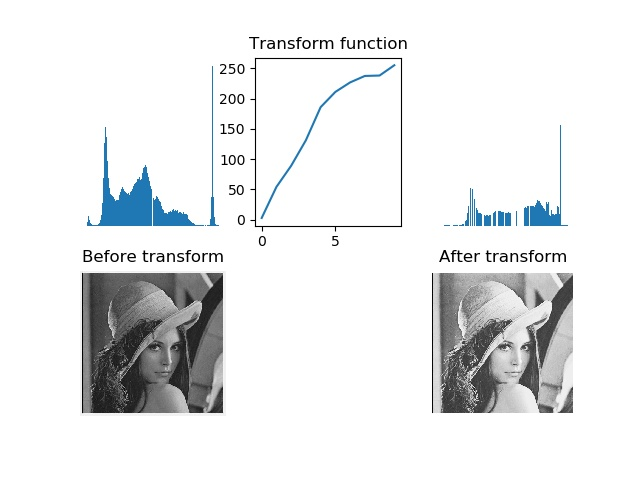Visible histogram equalization enhances the contrast of the image, the original image Byway gray area becomes clear.
PCA (Principal Component Analysis, Principal Component Analysis) is a very useful dimension reduction technique, it can be under the premise of using as little number of dimensions, keeping as much information training data. See details and use my other article: PCA dimensionality reduction

SciPy Numpy is built based on open source tools for numerical computation of the package. Scipy offers many efficient operation, can achieve numerical integration, optimization, statistics, signal processing, and for us the most important image processing functions.

Gaussian blur image is very classic image convolution example. In essence, it is to blur the image (grayscale) images \ (the I \) a Gaussian kernel and convolving operations:
\ [
I_ \ sigma = I * G_ \ sigma
\]
Wherein \ (* \) represents a convolution operation; \ (G \) represents the standard deviation \ (\ Sigma \) a two-dimensional Gaussian kernel, is defined as:
\ [
G_ \ sigma = \ frac {1} {2 \ pi \ sigma ^ 2} e ^ {- (x ^ 2 + y ^ 2) / 2 \ sigma ^ 2}
\]
Gaussian blur usually other part of the image processing operations, such as image interpolation operation, points of interest and other computing applications.

Useful filtering operations do Scipy scipy.ndimage.filters module. The module uses a one-dimensional fast way to calculate the convolution isolated. Use:

``````from PIL import Image
import numpy as np
from scipy.ndimage import filters

img = Image.open(r"girl.jpg").convert('L')
img = np.array(img)
img2 = filters.gaussian_filter(img, 2)
img3 = filters.gaussian_filter(img, 5)
img4 = filters.gaussian_filter(img, 10)``````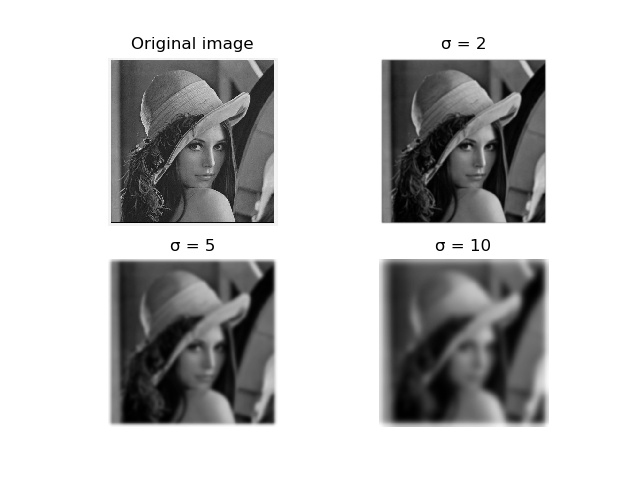Used above gaussian_filter () function in the parameter represents a standard deviation \ (\ Sigma \), seen as (\ Sigma \) increased \, the image becomes increasingly blurred. \ (\ Sigma \) greater image detail is lost after processing more. If you are going to blur a color image, simply for each color channel Gaussian blur:

``````from PIL import Image
import numpy as np
from scipy.ndimage import filters

img = Image.open(r"girl.jpg")
img = np.array(img)
img2 = np.zeros(img.shape)
for i in range(img2.shape):
img2[:, :, i] = filters.gaussian_filter(img[:, :, i], 5)
# 将像素值用八位表示
img2 = np.array(img2, 'uint8')``````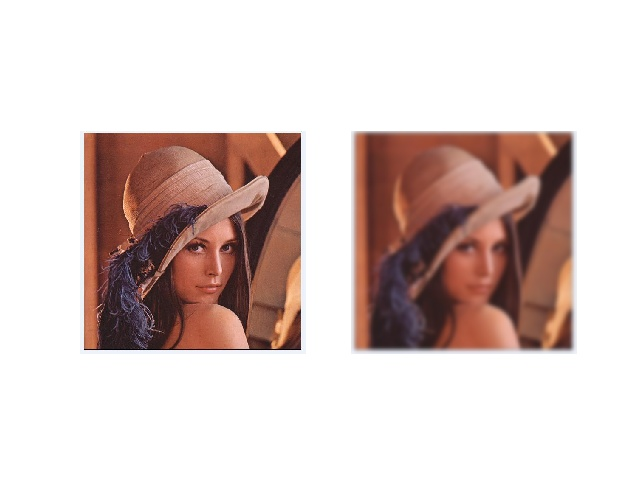In many applications, changes in the image intensity is very important, changes in intensity can be used grayscale image \ (X \) and \ (Y \) direction derivative \ (I_x \) and \ (I_y \) described

Gradient vector of the image is \ (\ bigtriangledown I = [I_x, I_y] ^ T \). Gradient has two important attributes, one of gradient size:
\ [
| \ Bigtriangledown I | = \ sqrt {I_x ^ 2 + I_y ^ 2}
\]
It describes the strength of the image intensity variations, the other angle of an image is:
\ [
\ Alpha = arctan2 (I_x, I_y)
\]
It describes the maximum image intensity at each point change direction. arctan2 Numpy in () function returns the signed angle in radians, the angle variation range for the \ ((- \ pi, \ pi) \)
The derivative can be calculated using a discrete approximation image manner. Most of the inverse image can be easily achieved by convolution:
\ [
I_x = I * D_x and I_y = I * D_y
\]
For \ (D_X \) and \ (D_y \), usually selected Prewitt filter:
\ [
D_x = \ left [
\ Begin {matrix}
1 & & \\ 0 -1
1 & & \\ 0 -1
-1 & 0 & 1
\ End {matrix}
\ Right]
\]
with
\ [
D_y = \ left [
\ Begin {matrix}
& -1 -1 -1 & \\
0 & 0 & 0 \\
1 & 1 & 1
\ End {matrix}
\ Right]
\]
Sobel filter or
\ [
D_x = \ left [
\ Begin {matrix}
1 & & \\ 0 -1
-2 0 & & \\ 2
-1 & 0 & 1
\ End {matrix}
\ Right]
\]
with
\ [
D_y = \ left [
\ Begin {matrix}
& -2 -1 -1 \\ &
0 & 0 & 0 \\
1 & 1 & 2
\ End {matrix}
\ Right]
\]
These derivative filter may be used to scipy.ndimage.filters standard convolution module simply achieved

``````from PIL import Image
import numpy as np
from scipy.ndimage import filters

img = Image.open(r"girl.jpg").convert('L')
img = np.array(img)
imgx = np.zeros(img.shape)
# Sobel导数滤波器
filters.sobel(img, 1, imgx)

imgy = np.zeros(img.shape)
filters.sobel(img, 0, imgy)

magnitude = np.sqrt(imgx**2+imgy**2)``````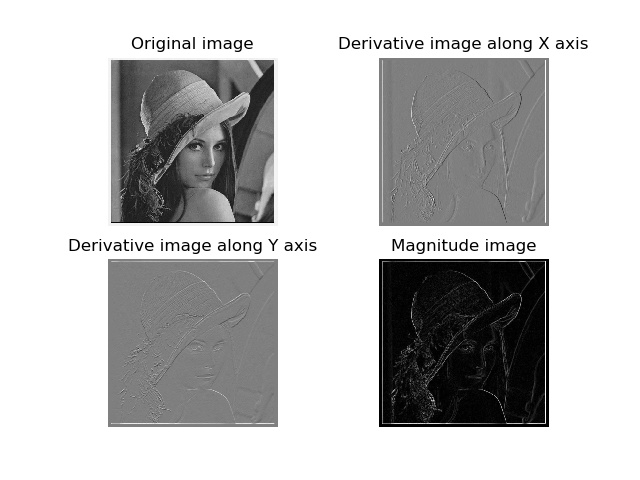Sobel () function to select the second parameter \ (X \) or a derivative \ (Y \) direction, the third output variable parameters are saved. In the image, the positive derivative as a bright pixel, the negative derivative as a dark pixel, a gray area represents the value of the derivative approaches zero.

Defective derivative images calculated above: In this method, the filter need to scale the image resolution varies varies (?). To be more robust in terms of image noise, and the derivative calculation on any scale, we can use a Gaussian derivative filters:
\ [
I_x = I * G _ {\ sigma x} and I_y = I * G _ {\ sigma y}
\]
Wherein, \ (G _ {\ sigma x} \) and \ (G _ {\ sigma y} \) represents \ derivative on \ (X \) and \ (Y \) direction (G_ \ sigma \), \ ( G_ \ sigma \) represents the standard deviation \ (\ Sigma \) Gaussian function. We use examples given below:

``````from PIL import Image
import matplotlib.pyplot as plt
import numpy as np
from scipy.ndimage import filters

img = Image.open(r"girl.jpg").convert('L')
img = np.array(img)

sigma = 2
imgx = np.zeros(img.shape)
imgy = np.zeros(img.shape)
filters.gaussian_filter(img, (sigma, sigma), (0, 1), imgx)
filters.gaussian_filter(img, (sigma, sigma), (1, 0), imgy)

magnitude = np.sqrt(imgx**2+imgy**2)``````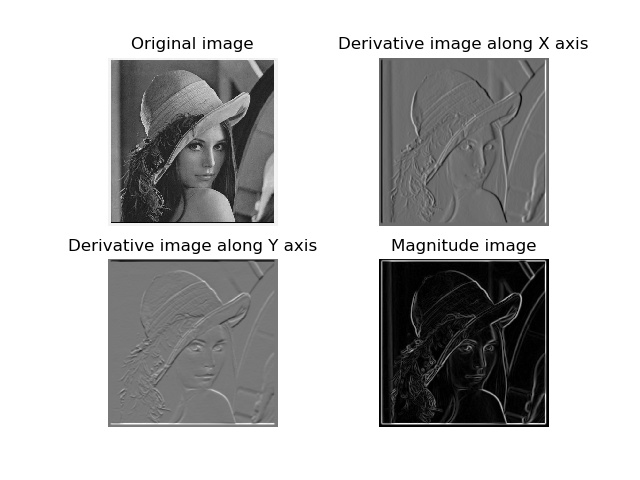When the image processing, noise removal is also a very important part. Denoising is while removing image noise, and image detail as possible structurally processing techniques, are given below ROF denoising model using the Demo:

``````from PIL import Image
import matplotlib.pyplot as plt
import numpy as np
from scipy.ndimage import filters

def de_noise(img, U_init, tolerance=0.1, tau=0.125, tv_weight=100):
U = U_init
Px = Py = img
error = 1
while error > tolerance:
Uold = U
# 变量U梯度的x分量
# 变量U梯度的y分量

# 更新对偶变量
NormNew = np.maximum(1, np.sqrt(PxNew**2+PyNew**2))

# 更新x,y分量
Px = PxNew / NormNew
Py = PyNew / NormNew

# 更新原始变量
RxPx = np.roll(Px, 1, axis=1)  # 将x分量向x轴正方向平移
RyPy = np.roll(Py, 1, axis=0)  # 将y分量向y轴正方向平移

DivP = (Px - RxPx) + (Py - RyPy)  # 对偶域散度
U = img + tv_weight * DivP

error = np.linalg.norm(U - Uold)/np.sqrt(img.shape * img.shape)

return U, img-U

if __name__ == '__main__':
im = np.zeros((500, 500))
im[100:400,100:400] = 128
im[200:300, 200:300] = 255
im = im + 30 * np.random.standard_normal((500, 500))

U, T = de_noise(im, im)
G = filters.gaussian_filter(im, 10)
plt.figure()
plt.gray()
plt.subplot(221).set_title("Original image")
plt.axis('off')
plt.imshow(im)
plt.subplot(222).set_title("Gauss blurred image")
plt.axis('off')
plt.imshow(G)
plt.subplot(223).set_title("ROF")
plt.axis('off')
plt.imshow(U)
plt.savefig('tmp.jpg')
plt.show()
``````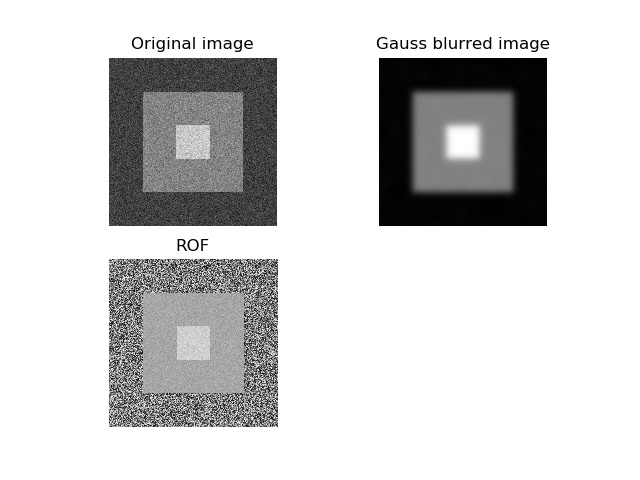After ROF denoised image edges and preserves the structural information of the image, while blurring the “noise.”

np.roll () function can cycle through elements, np.linalg.norm () is used to measure the difference between the two arrays.

After the free will complement the image de-noising

Reference books
Python Computer Vision